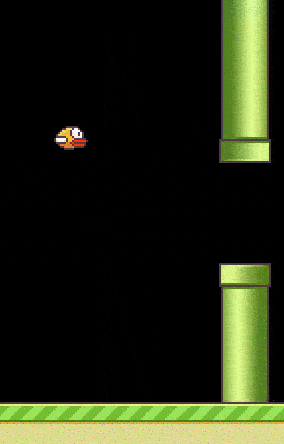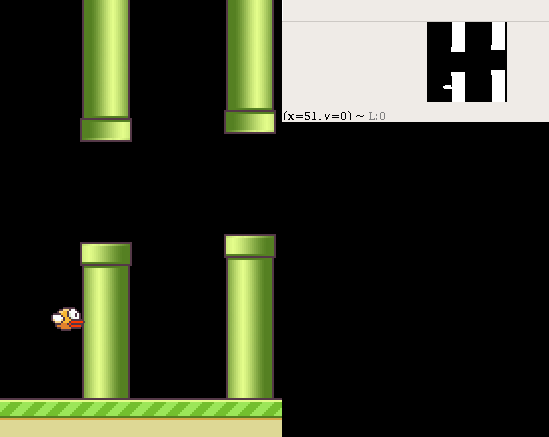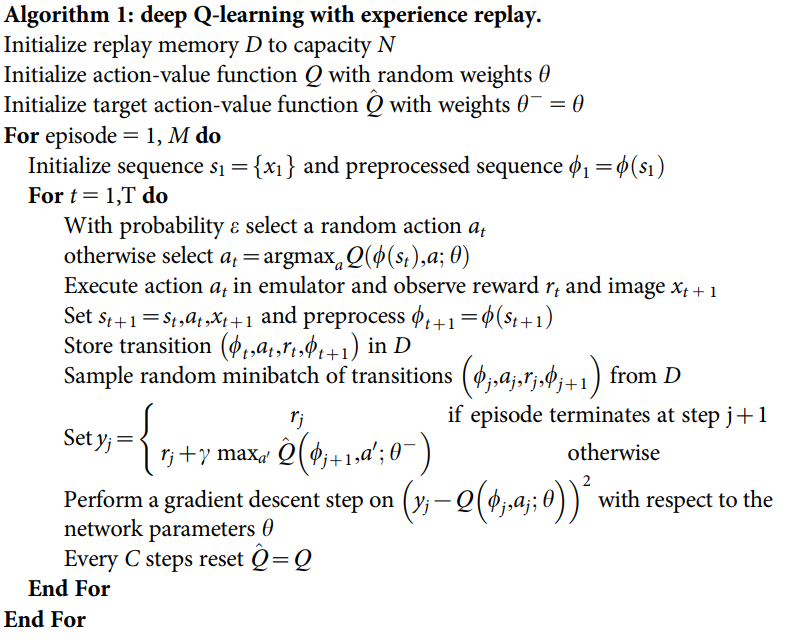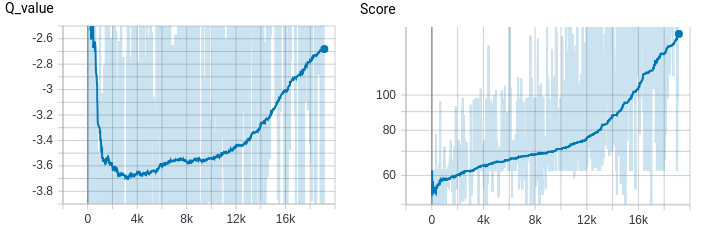# It's Really Easy To Play Flappy Bird Via Reinforcement LearningFlappy Bird game played by reinforcement learning algorithm (DQN) after training 18 hours

## Introduction

I think you must have heared about the famous game known as Flappy Bird. If not, please try it first. It’s not easy to get high score. However, for a computer, it’s maybe very simlpe!

In this post, I’ll show you how to play this game via reinforcement learning in only 180 lines of code!. Since the algorithm is introduced in this post: How To Play Cart Pole Game with Temporal Difference Learning By Pytorch?, I’ll mainly introduce how to train the computer to play the game instead of math.

The code is writen in python and the deep learning library is pytorch.

## Flappy Bird

We choose pygame learning environment for game rendering environment. First you need to install it, please check official site.

After installing the environment, you need to include the path to the PLE using

import sys
sys.path.append("/path/to/PyGame-Learning-Environment")


For example, if you use the code below, you can see the flapy bird!

import sys
sys.path.append("/path/to/PyGame-Learning-Environment")
from ple.games.flappybird import FlappyBird
from ple import PLE

game = FlappyBird()
p = PLE(game, fps=30, display_screen=True, force_fps=False)
p.init()
actions = p.getActionSet()
action_dict = {0: actions, 1:actions}

reward = 0.0

for i in range(10000):
if p.game_over():
p.reset_game()

state = p.getScreenRGB()
action = 1
reward = p.act(action_dict[action])


The state in this game can be RGB image returned by getScreenRGB() or non-visual state representation of the game returned by getGameState(). In this post, I will show you how to use convolutional neural networks to train the computer, thus we choose getScreenRGB(). The returned image size is (512, 288, 3), since the ground is useless we will remove them, and resize the image to 80x80, then binarize it for faster convergence. Here is the image preprecess function.

def img_preprocess(img):
img = cv2.rotate(img, cv2.ROTATE_90_CLOCKWISE)
img = cv2.flip(img,1)
img = cv2.cvtColor(img, cv2.COLOR_BGR2RGB)

img = img[:400,:]
img = cv2.resize(img, (80, 80))
img = cv2.cvtColor(img,cv2.COLOR_BGR2GRAY)
retval, img = cv2.threshold(img, 50, 255, cv2.THRESH_BINARY)
#img = torch.FloatTensor(img)
return img


With the image preprocessed, the image becomes:Image preprocess

## DQN

It’s a very simple convolutional neural networks writen in pytorch.

class DQN(torch.nn.Module):
def __init__(self, action_space):
super(DQN, self).__init__()
self.conv1 = nn.Sequential(nn.Conv2d(1, 32, kernel_size=8, stride=4), nn.ReLU(inplace=True))
self.conv2 = nn.Sequential(nn.Conv2d(32, 64, kernel_size=4, stride=2), nn.ReLU(inplace=True))
self.conv3 = nn.Sequential(nn.Conv2d(64, 64, kernel_size=3, stride=1), nn.ReLU(inplace=True))
self.fc1 = nn.Sequential(nn.Linear(6 * 6 * 64, 512), nn.ReLU(inplace=True))
self.fc2 = nn.Linear(512, action_space)

def forward(self, observation):
output = self.conv1(observation)
output = self.conv2(output)
output = self.conv3(output)
output = output.view(output.size(0), -1)
output = self.fc1(output)
output = self.fc2(output)
return output


Here is the algorithm from nature paper. The training code is respongding with the algorithm, please make sure to read the code and algorithm carefully.Deep Q-learning with experience replay

## Show me the code

Here is th full script.

import sys
sys.path.append("/path/to/PyGame-Learning-Environment")
from ple.games.flappybird import FlappyBird
from ple import PLE
import numpy as np
import random
from collections import deque
import torch
import torch.nn as nn
import torch.optim as optim
import copy
import cv2
from torch.utils.tensorboard import SummaryWriter

GAMMA = 0.99
LEARNING_RATE = 3e-6
MEMORY_SIZE = 50000
BATCH_SIZE = 48
EXPLORATION_MAX = 5e-2
EXPLORATION_MIN = 1e-5
EXPLORATION_DECAY = 0.9999

Model_path = "./model.pth"
writer = SummaryWriter()

class DQN(torch.nn.Module):
def __init__(self, action_space):
super(DQN, self).__init__()
self.conv1 = nn.Sequential(nn.Conv2d(1, 32, kernel_size=8, stride=4), nn.ReLU(inplace=True))
self.conv2 = nn.Sequential(nn.Conv2d(32, 64, kernel_size=4, stride=2), nn.ReLU(inplace=True))
self.conv3 = nn.Sequential(nn.Conv2d(64, 64, kernel_size=3, stride=1), nn.ReLU(inplace=True))
self.fc1 = nn.Sequential(nn.Linear(6 * 6 * 64, 512), nn.ReLU(inplace=True))
self.fc2 = nn.Linear(512, action_space)

def forward(self, observation):
output = self.conv1(observation)
output = self.conv2(output)
output = self.conv3(output)
output = output.view(output.size(0), -1)
output = self.fc1(output)
output = self.fc2(output)
return output
class GameSolver:
def __init__(self, action_space):
self.exploration_rate = EXPLORATION_MAX

self.action_space = action_space
self.memory = deque(maxlen=MEMORY_SIZE)
self.Q_policy = DQN(action_space)
self.Q_target = copy.deepcopy(self.Q_policy)
if torch.cuda.is_available():
self.Q_policy = self.Q_policy.cuda()
self.Q_target = self.Q_target.cuda()
self.optimizer = optim.Adam(self.Q_policy.parameters(), lr = LEARNING_RATE)
self.lossFuc = torch.nn.MSELoss()

def remember(self, state, action, reward, next_state, done):
self.memory.append((state, action, reward, next_state, done))

def predict(self, state):

q_values = self.Q_policy(state)

# best action
if np.random.rand() < self.exploration_rate:
max_q_index = [random.randrange(self.action_space)]
max_q_one_hot =  one_hot_embedding(max_q_index, self.action_space)
else:
max_q_index =  [torch.max(q_values, 0).indices.cpu().numpy().tolist()]
max_q_one_hot =  one_hot_embedding(max_q_index, self.action_space)
# print(torch.max(q_values).detach().numpy())
return max_q_index, max_q_one_hot, q_values

def experince_replay(self):
if len(self.memory) < BATCH_SIZE:
return
batch = random.sample(self.memory, BATCH_SIZE)
state_batch, action_batch, reward_batch, next_state_batch, terminal_batch = zip(*batch)
state_batch = torch.cat(tuple(state for state in state_batch))
action_batch = torch.cat(tuple(action for action in action_batch))
reward_batch = torch.cat(tuple(reward for reward in reward_batch))
reward_batch = reward_batch.view(len(reward_batch), 1)
next_state_batch = torch.cat(tuple(next_state for next_state in next_state_batch))
current_prediction_batch = self.Q_policy(state_batch)
next_prediction_batch = self.Q_target(next_state_batch)

y_batch = torch.cat(
tuple(reward if terminal else reward + GAMMA * torch.max(prediction) for reward, terminal, prediction in
zip(reward_batch, terminal_batch, next_prediction_batch)))

q_value = torch.sum(current_prediction_batch * action_batch, dim=1)
# y_batch = y_batch.detach()
loss = self.lossFuc(q_value, y_batch)
loss.backward()
self.optimizer.step()

self.exploration_rate *= EXPLORATION_DECAY
self.exploration_rate  = max(EXPLORATION_MIN, self.exploration_rate)

def Flappy_bird():
game = FlappyBird()
game.allowed_fps = None
p = PLE(game, fps=200, display_screen=True, force_fps=False)
p.init()
actions = p.getActionSet()
action_dict = {0: actions, 1:actions}
reward = 0.0
gameSolver = GameSolver(len(actions))
run = 0
while True:
run += 1
p.reset_game()
state = img_preprocess(p.getScreenRGB())
step = 0
if run % 5000 == 4999:
torch.save(gameSolver.Q_policy.state_dict(), Model_path)
while True:
step += 1
action, action_one_hot, p_values = gameSolver.predict(state)
reward = p.act(action_dict[action])
state_next = img_preprocess(p.getScreenRGB())
terminal = p.game_over()

reward = torch.tensor([reward])
if torch.cuda.is_available():
reward = reward.cuda()
action_one_hot = action_one_hot.cuda()
gameSolver.remember(state, action_one_hot, reward, state_next,
terminal)
state = state_next

if terminal:
print ("Run: " + str(run) + ", exploration: " + str(gameSolver.exploration_rate) + ", score: " + str(step) )
break
gameSolver.experince_replay()
if step % 50 == 0:
def one_hot_embedding(labels, num_classes):
"""Embedding labels to one-hot form.
Args:
labels: (LongTensor) class labels, sized [N,].
num_classes: (int) number of classes.

Returns:
(tensor) encoded labels, sized [N, #classes].
"""
y = torch.eye(num_classes)
return y[labels]

def img_preprocess(img):
img = cv2.rotate(img, cv2.ROTATE_90_CLOCKWISE)
img = cv2.flip(img,1)
img = cv2.cvtColor(img, cv2.COLOR_BGR2RGB)
img = img[:400,:]
img = cv2.resize(img, (80, 80))
img = cv2.cvtColor(img,cv2.COLOR_BGR2GRAY)
retval, img = cv2.threshold(img, 50, 255, cv2.THRESH_BINARY)
img = torch.FloatTensor(img)
img = img.unsqueeze(0)
img = img.unsqueeze(0)
if torch.cuda.is_available():
img = img.cuda()
return img

if __name__ == "__main__":
Flappy_bird()


Here is the result after training 18 hours:Deep Q-learning with experience replay

## Summary

In this post we introduced how to use neural networks to play Flappy Bird game in only 180 lines of code. However, as you can see, the system need a lot of time to learn even for such an easy game! Also, the DQN is not very stable since it will also make many mistakes. In the following posts, we will introduce other methods to improve DQN, and policy gradient methods that can be better than simple DQN.

Welcome to share or comment on this post: